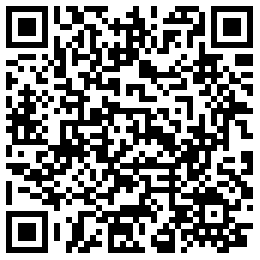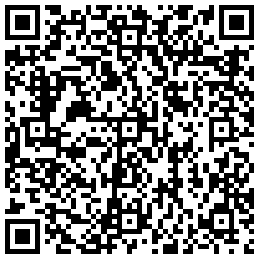# PHP基本语法详解

### PHP标记

PHP标记中有4中不同的风格供我们使用

<?php      ?>

#### *简短风格

<?     ?>

*SCRIPT风格

<script language=“php”>
echo (“这是类似 JavaScript 及 VBScript 语法 的 PHP 语言嵌入范例，指定脚本语言的方式”);
</script>

*ASP风格

<% echo (“ASP的风格的标记”); %>

PHP输出语句

#### PHP注释

``````对于阅读代码的人来说，注释其实就相当于代码的解释和说明。注释可以用来解释脚本的用途，脚本编写人，为什么要按如此的方法编写代码，上一次的修改时间等等，PHP支持C,C++和SHELL脚本风格的注释。

<?php
echo "这是第一种例子。\n"; // 本例是 C++ 语法的注释
/* 本例采用多行的
注释方式      */
echo "这是第两种例子。\n";
echo "这是第三种例子。\n"; # 本例使用 UNIX Shell 语法注释
?>
C 风格的注释在碰到第一个 */ 时结束。小心不要嵌套 C 风格的注释，当注释大量代码时，可能犯该错误。
<?php
/*
echo "This is a test"; /* This comment will cause a problem */
*/
?>``````

#### PHP数据类型

• boolean（布尔型）:表达了真值，可以为 TRUEFALSE

``````<?php
\$foo = True; // assign the value TRUE to \$foo
?> ``````

• 布尔值 FALSE
• 整型值 0（零）
• 浮点型值 0.0（零）
• 空白字符串和字符串 "0"
• 没有成员变量的数组
• 特殊类型 NULL（包括尚未设定的变量）

• integer（整型）

integer 为整数型别。在 32 位的操作系统中，它的有效范围是 -2,147,483,648 到 +2,147,483,647 (2^31+符号位)。要使用 16 进位整数可以在面加 0x。 整型值可以用十进制，十六进制或八进制符号指定，前面可以加上可选的符号（- 或者 +）。

``````<?php
\$a = 1234; // 十进制数
\$a = -123; // 一个负数
\$a = 0123; // 八进制数（等于十进制的 83）
\$a = 0x1A; // 十六进制数（等于十进制的 26）
?>``````
• float（也叫浮点数，双精度数或实数）

``````<?php
\$a = 1.234;
\$a = 1.2e3;
\$a = 7E-10;
?>``````
• string（字符串）

``````\$s = "I' m a student";
\$s = ' I am a “student” ';

"Why doesn't "this" work?"

实际上被PHP语法分析器分成三个部分:

"Why doesn't "——包含一个单引号的双引号串
this——多余的字符，分析器无法处理
" work?" ——普通字符串 ``````

``````Why doesn't \"that\" work?"

\$first_name = 'A';
\$last_name = 'B';
\$full_name = \$first_name . ' ' . \$last_name;

echo \$aa. ‘test<br/>’；
echo "\$aa  test<br/>"；//在双引号中，变量名称被变量值替代
echo ’\$aa  test<br/>’;；?>//在单引号中，变量名称或其他的文本都会不经修改而发送给浏览器``````

• array（数组）

array 为数组变量，可以是二维、三维或者多维数组，其中的元素也很自由，可以是 string、integer 或者 double，甚至是 array。 可以用 array() 语言结构来新建一个 array。它接受一定数量用逗号分隔的 key => value 参数对。

key 只能是整数和字符串

``````\$a = array( 1 => 'one', 2 => 'two', 3 => 'three' );
\$a = array( 'one', 'two',  'three' );默认下标是从0开始

\$MyArray1 = array("a", "b", "c", "d");//一维数组
\$MyArray2 = array(
"地支" => array("子", "丑", "寅", "卯"),
"生肖" => array("鼠", "牛", "虎", "兔"),
"数字" => array(1, 2, 3, 4)
); //二维数组
<?php
\$arr = array(12 => true,"foo" => "bar",  false);

echo \$arr["foo"]; // bar
echo \$arr;    // 1
?>

<?php
\$MyArray1 = array("a", "b", "c", "d");
echo \$MyArray1;
print_r(\$MyArray1);
?>``````

``````<?php
\$foo = 10;   // \$foo is an integer
\$bar = (bool) \$foo;   // \$bar is a boolean
?> ``````

• (int)，(integer) - 转换成整型
• (bool)，(boolean) - 转换成布尔型
• (float)，(double)，(real) - 转换成浮点型
• (string) - 转换成字符串
• (array) - 转换成数组
• (object) - 转换成对象

PHP 中的类型强制转换和 C 中的非常像：在要转换的变量之前加上用括号括起来的目标类型。

``````•	(int),(integer)            - 转换成整型
•	(bool),(boolean)           - 转换成布尔型
•	(float),(double),(real)    - 转换成浮点型
•	(string)                   - 转换成字符串
•	(array)                    - 转换成数组
•	(object)                   - 转换成对象``````

1.强制转换为布尔值 (bool)|(boolean) 要明示地将一个值转换成 boolean，用 (bool) 或者 (boolean) 来强制转换。但是很多情况下不需要用强制转换，因为当运算符，函数或者流程控制需要一个 boolean 参数时，该值会被自动转换。

``````?php
var_dump((bool) ""); // bool(false)
var_dump((bool) 1); // bool(true)
var_dump((bool) -2); // bool(true)
var_dump((bool) "foo"); // bool(true)
var_dump((bool) 2.3e5); // bool(true)
var_dump((bool) array(12)); // bool(true)
var_dump((bool) array()); // bool(false)
var_dump((bool) "false"); // bool(true)
?>``````

2.强制转换为整形(int)|(integer)

a.从bool转换
b.从浮点数转换     取整,超出范围,结果不定
c.从字符串转换     参看字符串转换为数值
d.从其它类型转换   先换成 bool值,再转换 决不要将未知的分数强制转换为 integer，这样有时会导致意外的结果。

``````<?php
echo (int) ( (0.1+0.7) * 10 ); // 显示 7
?>

\$str = "123.456abc7";  // (int)123
echo (int)\$str;
\$str = "abc123.456";   // (int)0
\$str = true;           // (int)1
\$str = false;          // (int)0``````

3.强制转换为浮点型 (int)|(double)|(real)|doubleval()|floatval()|intval()

4.强制换为字符串 (string) |strval()

• 布尔值 TRUE 将被转换为字符串 "1"，而值 FALSE 将被表示为 ""（即空字符串）。这样就可以随意地在布尔值和字符串之间进行比较。
• 整数或浮点数数值在转换成字符串时，字符串由表示这些数值的数字字符组成（浮点数还包含有指数部分）。
• 数组将被转换成字符串 "Array"，因此无法通过 echo() 或者 print() 函数来输出数组的内容。请参考下文以获取更多提示。
• 对象将被转换成字符串 "Object"。如果因为调试需要，需要将对象的成员变量打印出来，请阅读下文。如果希望得到该对象所依附的类的名称，请使用函数 get_class()。自 PHP 5 起，如果合适可以用 __toString() 方法。
• 资源类型总是以 "Resource id #1" 的格式被转换成字符串，其中 1 是 PHP 在运行时给资源指定的唯一标识。如果希望获取资源的类型，请使用函数 get_resource_type()。
• NULL 将被转换成空字符串。

4. 强制转换为数组 (array)

• 对于任何的类型：整型、浮点、字符串、布尔和资源，如果将一个值转换为数组，将得到一个仅有一个元素的数组（其下标为 0），该元素即为此标量的值。
• 如果将一个对象转换成一个数组，所得到的数组的元素为该对象的属性（成员变量），其键名为成员变量名。
• 如果将一个 NULL 值转换成数组，将得到一个空数组。

5. 转换为对象 (object)

6. 转换为资源 (无法转换)

■PHP 类型比较表(看手册附录P)

• 注意
• HTML 表单并不传递整数、浮点数或者布尔值，它们只传递字符串。要想检测一个字符串是不是数字，可以使用 is_numeric() 函数。

### PHP变量

`<strong>变量：</strong>`

PHP 是一门松散类型的语言（Loosely Typed Language

``````<?php \$a = 'name'；
\$\$a = '张三'；?>``````

``````<?php
\$a = "Hello";
\$\$a = "world";
echo "\$a, \$hello";   // Hello, world
echo "\$a, {\$a}";    // 也是 Hello, world
?>``````

作用域是指在一个脚本中某个变量在哪些地方可以使用或可见。

全局变量(global variable)

``````<?php
\$a = 1;\$b = 2;
function Sum(){
global \$a, \$b;
\$b = \$a + \$b;
}
Sum();
echo \$b;
?>    输出是’3’``````

`<strong>局部变量(</strong>`local variable`<strong>)</strong>：`任何用于函数内部的变量将被限制在局部函数范围内。

``````<?php
\$a = 1; /* global scope */
function Test(){
(\$a = 2 ;)
echo \$a; /* reference to local scope variable */
}
Test();
?>   什么也不输出``````

`<strong>静态变量(</strong>`static variable`<strong>)</strong>：`在函数退出时, 这个变量始终存在，不被销毁， 但不能被其它函数使用, 当再次进入该函数时, 将保存上次的结果。

``````<?php
// 静态变量的例子
function myfunc() {
static \$mystr;
\$mystr.="哈";
echo \$mystr."<br>\n";
}
myfunc();   // 哈
myfunc();   // 哈哈
myfunc();   // 哈哈哈
?>``````

### PHP运算符

（“/”）总是返回浮点数，即使两个运算数是整数（或由字符串转换成的整数）也是这样。

``````<?php
\$a = 8;
\$b = 2;
\$c = 3;
echo \$a+\$b."<br>\n";
echo \$a-\$b."<br>\n";
echo \$a*\$b."<br>\n";
echo \$a/\$b."<br>\n";
echo \$a%\$c."<br>\n";
?>``````

``````<?php
\$a = "Hello ";
\$b = \$a . "World!"; // now \$b contains "Hello World!"

\$a = "Hello ";
\$a .= "World!";     // now \$a contains "Hello World!"
?> ``````

``````<?php

\$a = (\$b = 4) + 5; // \$a 现在成了 9，而 \$b 成了 4。

?>``````

``````<?php
\$a = 5;
\$a += 2;    // 即 \$a = \$a + 2;
echo \$a."<br>\n";
\$b = "哇";
\$b .= "哈";   // \$b = "哇哈";
\$b .= "哈";   // \$b = "哇哈哈";
echo "\$b<br>\n";
?>``````

注: 递增／递减运算符不影响布尔值。递减 NULL 值也没有效果，但是递增 NULL 的结果是 1。注意字符变量只能递增，不能递减。

``````<?php
echo "**********";
\$a = 5;
echo "a++" . \$a++ . "<br />\n";
echo "a " . \$a . "<br />\n";

echo "**********";
\$a = 5;
echo "++a" . ++\$a . "<br />\n";
echo "a" . \$a . "<br />\n";

echo "**********";
\$a = 5;
echo "a--" . \$a-- . "<br />\n";
echo "a" . \$a . "<br />\n";

echo "**********";
\$a = 5;
echo "--a" . --\$a . "<br />\n";
echo "a" . \$a . "<br />\n";
?>``````

“与”和“或”有两种不同形式运算符的原因是它们运算的优先级不同

``````<?
\$a=0;
\$b=1;
if (\$a and \$b){
echo "真";
} else {
echo"假";
}
?>

<?
\$a="0";
\$b="1";
if (\$a and \$b){
echo "真";
} else {
echo"假";
}
?>``````

### PHP语句

`        第一种是只有用到 if 条件，当作单纯的判断。解释成 "若发生了某事则怎样处理"。`
``````if (expr) {
statement
}``````

``````<?php
if (1==1) echo "1等于1";
?> ``````

``````<?php
if (1==1) {
echo "1等于1";
echo "1就等于1";
}
?> ``````

``````<?php
\$a="1";
\$b="2";
if ( \$a==\$b){
echo "a等于b";
}
else{
echo"a不等于b";
}
?> ``````

``````<html>
<body>

<?php
\$d=date("D");
if (\$d=="Fri")
echo "周末愉快!";
else
echo "美好的一天!";
?>

</body>
</html>``````

``````<html>
<body>

<?php
\$d=date("D");
if (\$d=="Fri")
{
echo "Hello!<br />";
echo "周末愉快!";
echo "星期一见!";
}
?>

</body>
</html>``````

``````<?php
if (\$a > \$b) {
echo "a 比 b 大";
} elseif (\$a == \$b) {
echo  "a 等于 b";
} else {
echo "a 比 b 小";
}
?> ``````

``````<html>
<body>

<?php
\$d=date("D");
if (\$d=="Fri")
echo "周末愉快!";
elseif (\$d=="Sun")
echo "星期天愉快!";
else
echo "美好的一天!";
?>

</body>
</html>``````

do..while 是重复叙述的循环，可以分成两种模式。

while (expr) {

statement

}

``````<?php
\$i = 1;
while (\$i <= 10) {
print \$i++;
echo ". 以后不敢了<br>\n";
}
?>``````

while 可以不用大括号来包住执行部分，而使用冒号加上 endwhile。见下例

``````<?php
\$i = 1;
while (\$i <= 10):
print \$i++;
echo ". 以后不敢了<br>\n";
endwhile;
?>``````

for 循环就单纯只有一种，没有变化，它的语法如下

``````for (expr1; expr2; expr3) {
statement
}``````

``````<?php
for (\$i=1; \$i<=10; \$i++) {
echo "\$i. 以后不敢了<br>\n";
}
?>``````

switch 循环，通常处理复合式的条件判断，每个子条件，都是 case 指令部分。在实作上若使用许多类似的 if 指令，可以将它综合成 switch 循环。

``````switch (expr) {
case expr1:
statement1;
break;
case expr2:
statement2;
break;
:
:
default:
statementN;
break;
}``````

``````<?php
switch (date("D")) {
case "Mon":
echo "今天星期一";
break;
case "Tue":
echo "今天星期二";
break;
case "Wed":
echo "今天星期三";
break;
case "Thu":
echo "今天星期四";
break;
case "Fri":
echo "今天星期五";
break;
default:
echo "今天放假";
break;
}
?>``````

••